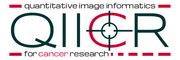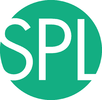# Documentation/Nightly/Modules/DWModeling

Home < Documentation < Nightly < Modules < DWModeling
 For the stable Slicer documentation, visit the 4.10 page.

# Introduction and Acknowledgements

 Extension: SlicerProstate Acknowledgments: This work supported in part the National Institutes of Health, National Cancer Institute through the following grants: Quantitative MRI of prostate cancer as a biomarker and guide for treatment, Quantitative Imaging Network (U01 CA151261, PI Fennessy) Enabling technologies for MRI-guided prostate interventions (R01 CA111288, PI Tempany) The National Center for Image-Guided Therapy (P41 EB015898, PI Tempany) Quantitative Image Informatics for Cancer Research (QIICR) (U24 CA180918, PIs Kikinis and Fedorov). Authors: Andrey Fedorov (SPL), Alireza Mehrtash (SPL) Contact: Andrey Fedorov, fedorov@bwh.harvard.edu License: Slicer LicenseNational Center for Image Guided Therapy (NCIGT)Quantitative Image Informatics for Cancer ResearchSurgical Planning Laboratory (SPL)

# Module Description

The purpose of this module is to provide estimation of DWI quantitative parameters using commonly diffusion models. Input data should be a trace DWI image correctly identified as a multivolume by b-value by the Slicer DICOMBrowser. The module currently supports mono-exponential, bi-exponential and kurtosis DW models, see  for further details on the models.

Modeling results can be explored (interactive curve plotting of the input and fitted data) using MultiVolumeExplorer module.

Development of this module was motivated by the application in DWI of prostate cancer. Testing was done on the DWI MRI data collected on a 3T GE Discovery 750w platform (trace images from DWI obtained with 3 orthogonal diffusion directions) at Brigham and Women's hospital. The module was used to obtain results presented in .

# Use Cases

• tissue characterization from DWI MRI
• quantitative image analysis
• treatment response assessment

# Tutorials

None at this time ... stay tuned!

# Panels and their use

Input

• Input image: multivolume node containing multi-b-value trace image loaded using DICOM module
• Model: mono-, bi-exponential or kurtosis model should be selected
• Input mask: segmentation of the region of interest (optional); if specified, model fitting will be performed only within the specified mask
• B-values to include: list of b-values that should be used in the fitting process (optional); this parameter is used only if not empty
• B-values to exclude: list of b-values that should NOT be used in the fitting process (optional); this parameter is used only if not empty

Output common to all models

• Fitted volume: (output) multi-volume containing the fitted model sampled at the b-values of the input dataset
• Quality of fit volume: (optional) R-squared

Model-specific outputs

• MonoExponential model output
• MonoExp diffusion map
• BiExponential model outputs
• Slow diffusion map
• Fast diffusion map
• Fast diffusion fraction map
• Kurtosis model outputs
• Kurtosis diffusion map
• Kurtosis map

Parameters:

• Input: Input parameters
• Input image (imageName): Input DWI MRI trace multivolume image
• Model (modelName): Select the mathematical model used to fit the data
• Input mask (maskName): Input mask. Optional; if not specified, fitting will be done at all voxels.
• B-values to include (bValuesToInclude): List of integers corresponding to the b-values that should be included in fitting data. Note that only one of the two lists (inlusion or exclusion) should be populated, or both can be empty, in which case all b-values will be used. Optional; if not defined, all b-values will be used.
• B-values to exclude (bValuesToExclude): List of integers corresponding to the b-values that should be excluded from fitting data. Note that only one of the two lists (inlusion or exclusion) should be populated, or both can be empty, in which case all b-values will be used. Optional; if not defined, all b-values will be used.
• Output common to all models:
• Fitted volume (fittedVolumeFileName): Output volume containing the values of the fitted function
• R^2 quality of fit volume (rsqrVolumeFileName): Output volume containing the R^2 measure of the quality of fit. This measure is calculated only for the b-values used in the fitting process.
• SSD for the fitted b-values (ssdFittedVolumeFileName): Volume with the pixel-wise sum of squared differences (SSD) map that takes into consideration only those b-values that were used in the fitting process
• SSD for all b-values (ssdVolumeFileName): Volume with the pixel-wise sum of squared differences (SSD) map that takes into consideration all b-values. Note that this map will be identical to the previous one if the fitting procedure utilized all b-values
• Chi squared for the fitted b-values (csFittedVolumeFileName): Volume with the pixel-wise chi squared map that takes into consideration only those b-values that were used in the fitting process
• Chi squared for all b-values (csVolumeFileName): Volume with the pixel-wise pixel-wise chi squared map that takes into consideration all b-values. Note that this map will be identical to the previous one if the fitting procedure utilized all b-values
• MonoExponential model output:
• MonoExp diffusion map (adcMapFileName): Diffusion coefficient map of the mono-exponential model
• BiExponential model outputs:
• Slow diffusion map (slowDiffMapFileName): Slow diffusion coefficient map of the bi-exponential model
• Fast diffusion map (fastDiffMapFileName): Fast diffusion coefficient map of the bi-exponential model
• Fast diffusion fraction map (fastDiffFractionMapFileName): Fast diffusion fraction map of the bi-exponential model
• Kurtosis model outputs:
• Kurtosis diffusion map (kurtosisDiffMapFileName): Diffusion coefficient map of the kurtosis model
• Kurtosis map (kurtosisMapFileName): Kurtosos map
• Gamma distribution model outputs: See Oshio et al. 2014
• Theta parameter map (thetaMapFileName): Theta parameter of the gamma distribution model
• k map (kMapFileName): k map of the gamma distribution model
• Mode map (modeMapFileName): Mode map of the gamma distribution model ( (k-1)*theta )
• Stretched exponential model outputs: See Bennett et al. 2003
• DDC map (DDCMapFileName): Distributed Diffusion Coefficient map
• Stretching parameter map (alphaMapFileName): Stretching parameter (alpha) map
• Initialization: Model-specific initial parameters
• Mono-exponential model (monoExpInitParameters): List of initial model parameters in the following format (all numbers are floating point):initialScale,initialADC
• Bi-exponential model (biExpInitParameters): List of initial model parameters in the following format (all numbers are floating point):initialScale,initialFastDiffusionFraction,initialSlowDiffusionCoefficient,initialFastDiffusionCoefficient
• Kurtosis model (kurtosisInitParameters): List of initial model parameters in the following format (all numbers are floating point):initialScale,initialKurtosis,initialKurtosisDiffusion
• Stretched exponential model parameters (stretchedExpInitParameters): List of initial model parameters in the following format (all numbers are floating point):initialScale,initialDDC,initialAlpha
• Gamma distribution model (gammaInitParameters): List of initial model parameters in the following format (all numbers are floating point):initialScale,initialK,initialTheta

List of parameters generated transforming this XML file using this XSL file. To update the URL of the XML file, edit this page.